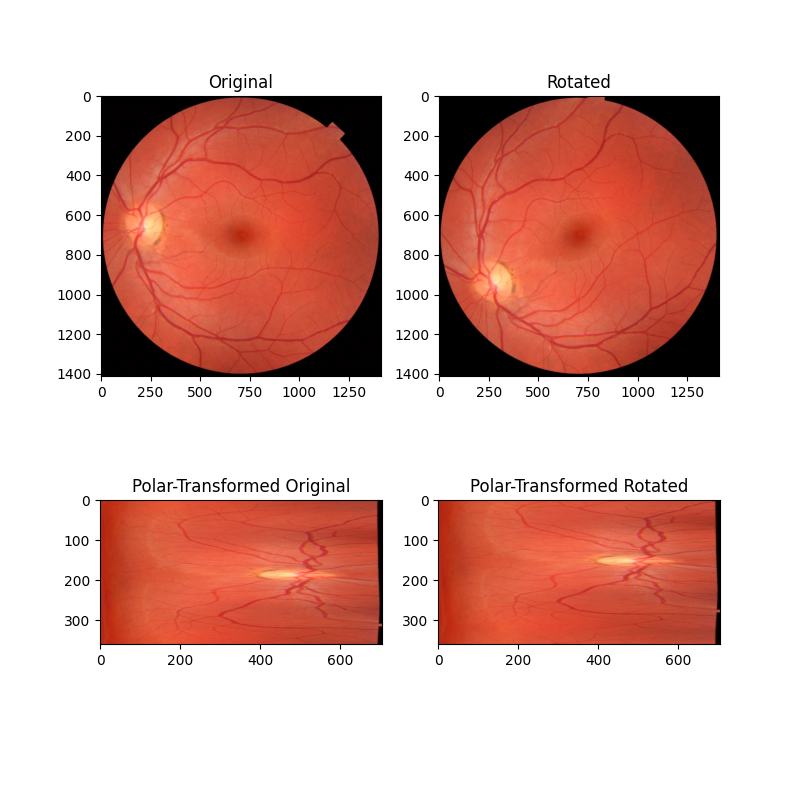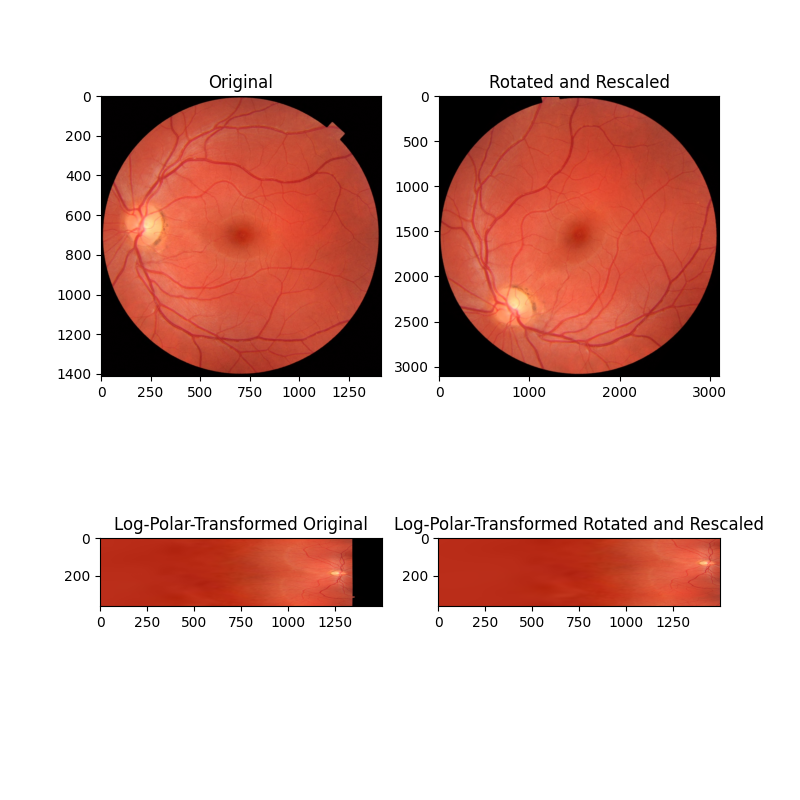# Polar and Log-Polar Transformations¶

Rotation differences between two images can be converted to translation differences along the angular coordinate ($$\theta$$) axis of the polar-transformed images. Scaling differences can be converted to translation differences along the radial coordinate ($$\rho$$) axis if it is first log transformed (i.e., $$\rho = \ln\sqrt{x^2 + y^2}$$). Thus, in this example, we use phase correlation (feature.register_translation) to recover rotation and scaling differences between two images that share a center point.

## Recover rotation difference with a polar transform¶

import numpy as np
import matplotlib.pyplot as plt

from skimage import data
from skimage.feature import register_translation
from skimage.transform import warp_polar, rotate
from skimage.util import img_as_float

angle = 35
image = data.retina()
image = img_as_float(image)
rotated = rotate(image, angle)

fig, axes = plt.subplots(2, 2, figsize=(8, 8))
ax = axes.ravel()
ax.set_title("Original")
ax.imshow(image)
ax.set_title("Rotated")
ax.imshow(rotated)
ax.set_title("Polar-Transformed Original")
ax.imshow(image_polar)
ax.set_title("Polar-Transformed Rotated")
ax.imshow(rotated_polar)
plt.show()

shifts, error, phasediff = register_translation(image_polar, rotated_polar)
print("Expected value for counterclockwise rotation in degrees: "
f"{angle}")
print("Recovered value for counterclockwise rotation: "
f"{shifts}")Out:

Expected value for counterclockwise rotation in degrees: 35
Recovered value for counterclockwise rotation: 35.0


## Recover rotation and scaling differences with log-polar transform¶

from skimage.transform import rescale

# radius must be large enough to capture useful info in larger image
angle = 53.7
scale = 2.2
image = data.retina()
image = img_as_float(image)
rotated = rotate(image, angle)
rescaled = rescale(rotated, scale, multichannel=True)
scaling='log', multichannel=True)
scaling='log', multichannel=True)

fig, axes = plt.subplots(2, 2, figsize=(8, 8))
ax = axes.ravel()
ax.set_title("Original")
ax.imshow(image)
ax.set_title("Rotated and Rescaled")
ax.imshow(rescaled)
ax.set_title("Log-Polar-Transformed Original")
ax.imshow(image_polar)
ax.set_title("Log-Polar-Transformed Rotated and Rescaled")
ax.imshow(rescaled_polar)
plt.show()

# setting upsample_factor can increase precision
tparams = register_translation(image_polar, rescaled_polar, upsample_factor=20)
shifts, error, phasediff = tparams
shiftr, shiftc = shifts[:2]

# Calculate scale factor from translation
shift_scale = 1 / (np.exp(shiftc / klog))

print(f"Expected value for cc rotation in degrees: {angle}")
print(f"Recovered value for cc rotation: {shiftr}")
print()
print(f"Expected value for scaling difference: {scale}")
print(f"Recovered value for scaling difference: {shift_scale}")Out:

Expected value for cc rotation in degrees: 53.7
Recovered value for cc rotation: 53.75

Expected value for scaling difference: 2.2
Recovered value for scaling difference: 2.1981889915232165


Total running time of the script: ( 0 minutes 6.876 seconds)

Gallery generated by Sphinx-Gallery